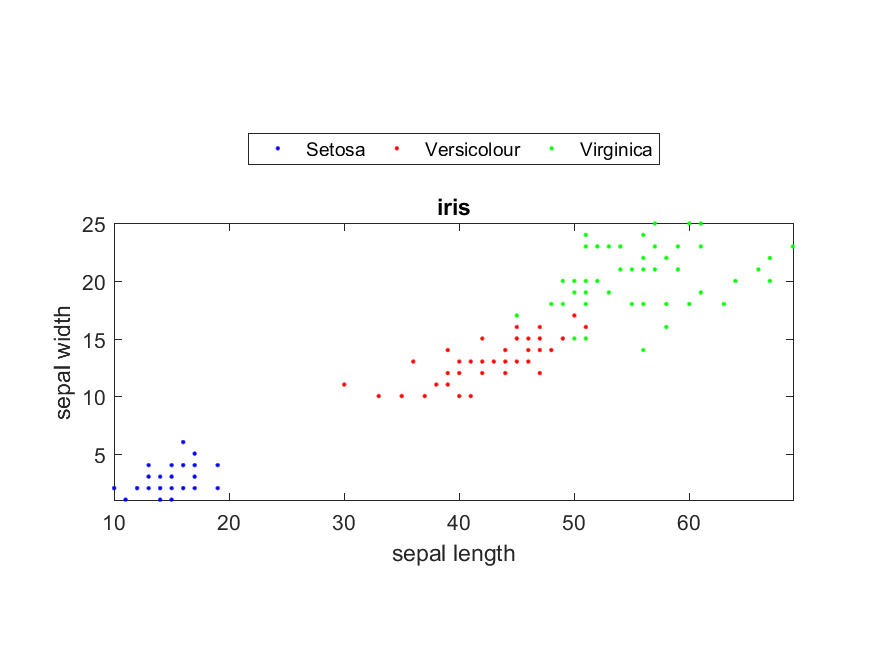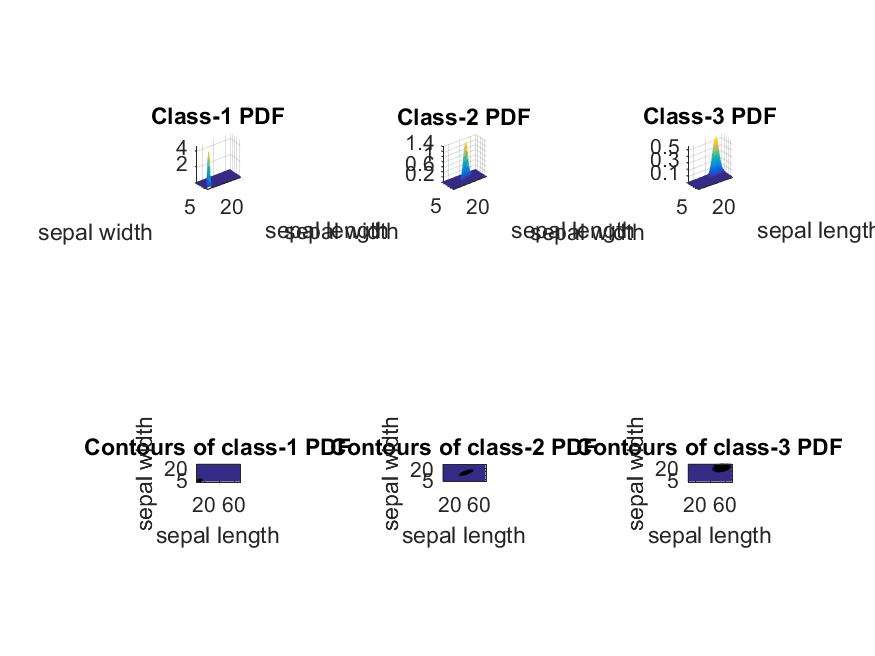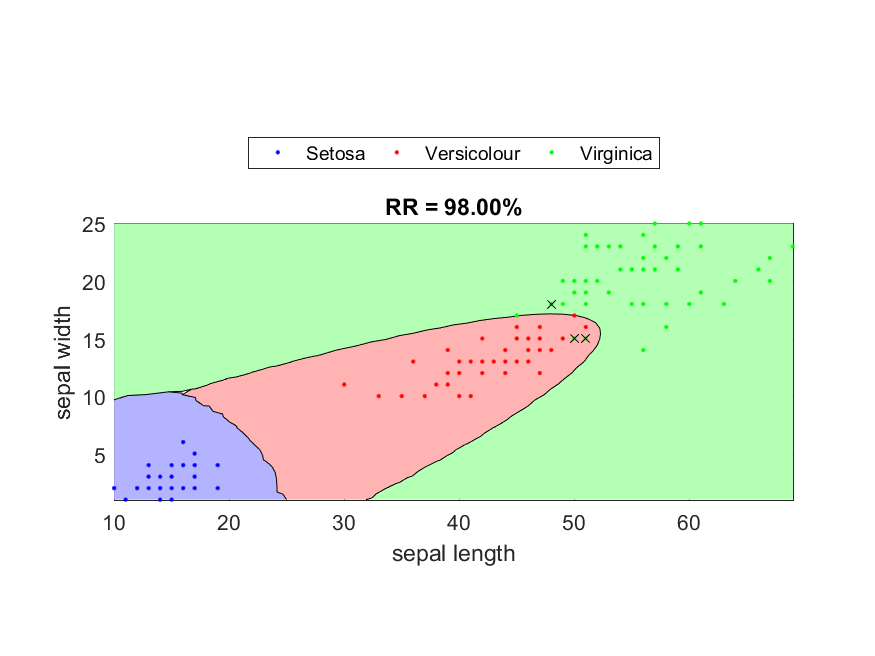[chinese][all]

If we assume that data entries in the same class of the training set are generated by a probability density function (PDF), we can use the concept of MLE (maximum likelihood estimate) to identify the parameters of the PDF. These PDFs (with each PDF for a class) can then be used to construct a classifier which can evaluate the likelihood for a data point being in a class. One of the commonly used PDFs is the d-dimensional Gaussian (normal) distribution: $$g(\mathbf{x}|\mathbf{\mu}, \Sigma)= \frac{1}{\sqrt{(2\pi)^d |\Sigma|}} exp\left(-\frac{1}{2}(\mathbf{x}-\mathbf{\mu})^T\Sigma^{-1}(\mathbf{x}-\mathbf{\mu})\right)$$ The resulting classifier is often called the quadratic classifier (QC) since the decision boundary between any two classes is a quadratic functions in the feature space. In contrast to the commonly used Gaussian mixture models (GMM for short), the quadratic classifier uses only one Gaussian distribution for each class.

The approach of the quadratic classifier can be summarized as follows:

1. Assume that data in class i is governed by a d-dimensional Gaussian probability density function: $$g(\mathbf{x}|\mathbf{\mu_i}, \Sigma_i)= \frac{1}{\sqrt{(2\pi)^d |\Sigma_i|}} exp\left(-\frac{1}{2}(\mathbf{x_i}-\mathbf{\mu_i})^T\Sigma_i^{-1}(\mathbf{x_i}-\mathbf{\mu_i})\right)$$ where mi is the mean vector and Si is the covariance matrix of this PDF, and |Si| is the determinant of Si. Given the training data {x1, x2, ... xn} in class i, the optimum parameters in the sense of maximum likelihood can be expressed as follows: $$\left\{ \begin{array}[rcl] \mathbf{\mu}_i & = & \frac{\mathbf{x}_1+\mathbf{x}_2+\cdots+\mathbf{x}_n}{n} \\ \Sigma_i & = & \frac{(\mathbf{x_1}-\mathbf{\mu}_i)(\mathbf{x_1}-\mathbf{\mu}_i)^T + (\mathbf{x_2}-\mathbf{\mu}_i)(\mathbf{x_2}-\mathbf{\mu}_i)^T + \cdots + (\mathbf{x_n}-\mathbf{\mu}_i)(\mathbf{x_n}-\mathbf{\mu}_i)^T}{n} \\ \end{array} \right.$$
2. Since the Gaussian PDF does not reflect the prior probability of class i (denoted by p(ci)), we usually use p(ci)g(x, mi, Si) to represent the probability density of a given vector x. In practice, p(ci) is computed as the number of entries of class i divided by the number of total entries in the training set.
3. In application, for a given vector x of unknown class, the higher the probability density p(ci)g(x, mi, Si) is, the more likelihood that this vector belongs to class i.

When implementing the classifier, usually we do not calculate p(ci)g(x, mi, Si) directly since the exponential function is likely to introduce round-off errors. Instead, we compute the natural logarithm of the probability density, as follows: $$\ln \left( p(c_i)g(\mathbf{x}|\mathbf{\mu}, \Sigma) \right) = \ln p(c_i) - \frac{d \ln(2\pi)+ \ln |\Sigma|}{2} - \frac{(\mathbf{x}-\mathbf{\mu})^T\Sigma^{-1}(\mathbf{x}-\mathbf{\mu})}{2}$$ The decision boundary between class i and j is represented by the following trajectory: $$p(c_i)g(\mathbf{x}|\mathbf{\mu}_i, \Sigma_i) = p(c_j)g(\mathbf{x}|\mathbf{\mu}_j, \Sigma_j)$$ Taking the logrithm of both sides, we have $$\ln p(c_i) - \frac{d \ln(2\pi)+ \ln |\Sigma_i|}{2} - \frac{(\mathbf{x}-\mathbf{\mu}_i)^T\Sigma_i^{-1}(\mathbf{x}-\mathbf{\mu}_i)}{2} = \ln p(c_j) - \frac{d \ln(2\pi)+ \ln |\Sigma_j|}{2} - \frac{(\mathbf{x}-\mathbf{\mu}_j)^T\Sigma_j^{-1}(\mathbf{x}-\mathbf{\mu}_j)}{2}$$ After simplification, we have the decision boundary as the following equation: $$2\ln p(c_i) + \ln |\Sigma_i| - (\mathbf{x}-\mathbf{\mu}_i)^T\Sigma_i^{-1}(\mathbf{x}-\mathbf{\mu}_i) = 2\ln p(c_j) + \ln |\Sigma_j| - (\mathbf{x}-\mathbf{\mu}_j)^T\Sigma_j^{-1}(\mathbf{x}-\mathbf{\mu}_j)$$ $$(\mathbf{x}-\mathbf{\mu}_i)^T\Sigma_i^{-1}(\mathbf{x}-\mathbf{\mu}_i) - (\mathbf{x}-\mathbf{\mu}_j)^T\Sigma_j^{-1}(\mathbf{x}-\mathbf{\mu}_j) = \ln \left( \frac{p^2(c_i)|\Sigma_i|}{p^2(c_j)|\Sigma_j|} \right)$$ where the right-hand side is a constant. Since both $(\mathbf{x}-\mathbf{\mu}_i)^T\Sigma_i^{-1}(\mathbf{x}-\mathbf{\mu}_i)$ and $(\mathbf{x}-\mathbf{\mu}_j)^T\Sigma_j^{-1}(\mathbf{x}-\mathbf{\mu}_j)$ are quadratic, the above equation represents a decision boundary of the quadratic form in the d-dimensional feature space.

In particular, if $\Sigma_i=\Sigma_j=\Sigma$, the decision boundary is reduced to a linear equation, as follows: $$\underbrace{(\mathbf{\mu}_i-\mathbf{\mu}_j) \Sigma}_{\mathbf{c}} \mathbf{x} = \underbrace{\mathbf{\mu}_i \Sigma \mathbf{\mu}_i - \mathbf{\mu}_j \Sigma \mathbf{\mu}_j - \ln \left( \frac{p^2(c_i)|\Sigma_i|}{p^2(c_j)|\Sigma_j|} \right)}_{constant} \Longrightarrow \mathbf{cx}=constant$$

In the following example, we shall use dimensions 3 and 4 of the Iris dataset for the quadratic classifier. First of all, we can plot the data distribution:

Example 1: qcDataPlot01.mDS = prData('iris'); DS.input=DS.input(3:4, :); % Only take dimensions 3 and 4 for 2d visualization dsScatterPlot(DS); % Scatter plot of the datasetThen we can use "qcTrain" for constructing a QC for classification:

Example 2: qcTrain01.mDS = prData('iris'); DS.input=DS.input(3:4, :); % Only take dimensions 3 and 4 for 2d visualization [qcPrm, logProb, recogRate, hitIndex]=qcTrain(DS); fprintf('Recog. rate = %f%%\n', recogRate*100);Recog. rate = 98.000000%

So for such a inside test, the recognition rate of QC is 98% using only features 3 and 4.

In the above example, each misclassified data point is labeled with a cross. Moreover, we use the number of entries of each class as the class prior probability for computing the probability density. In general, there are two ways to specify class prior probabilities:

• Use the number of entries in each class as the prior probabilities. You can divide the total number of entries in the training set to have a real probability between 0 and 1. In practice, the division does not change the classification results since it is applied to every class' PDF. (You can use the function classDataCount.m to find the number of entries in each class.)
• If we have the domain knowledge of the prior probabilities, we can assign them directly.

For the above example, we can go one step further to plot the Gaussian PDFs as 3D surfaces and display the corresponding contours, as follows:

Example 3: qcPlot01.mDS=prData('iris'); DS.input=DS.input(3:4, :); [qcPrm, logProb, recogRate, hitIndex]=qcTrain(DS); qcPlot(DS, qcPrm, '2dPdf');Based on the computed PDF for each class, we can plot the decision boundaries, as follows:

Example 4: qcPlot02.mDS=prData('iris'); DS.input=DS.input(3:4, :); [qcPrm, logProb, recogRate, hitIndex]=qcTrain(DS); DS.hitIndex=hitIndex; % Attach hitIndex to DS for plotting qcPlot(DS, qcPrm, 'decBoundary');As we have derived earlier, the decision boundaries should be quadratic functions in the 2D plane. The above plots do verify this fact.

If the class prior probabilities vary a lot among different classes, we might get seemingly wrong result by using the quadratic classifier. This problem is explored in the exercise.

We can also set the class prior probabilities according to the cost associated with false-positive and false-negative cases. This will be explained later.

If the data distribution is too complicated to model using a single Gaussian PDF for each class, then we have other choices such as multiple Gaussian classifiers or Gaussian mixture models, to be detailed later.

Data Clustering and Pattern Recognition (資料分群與樣式辨認)